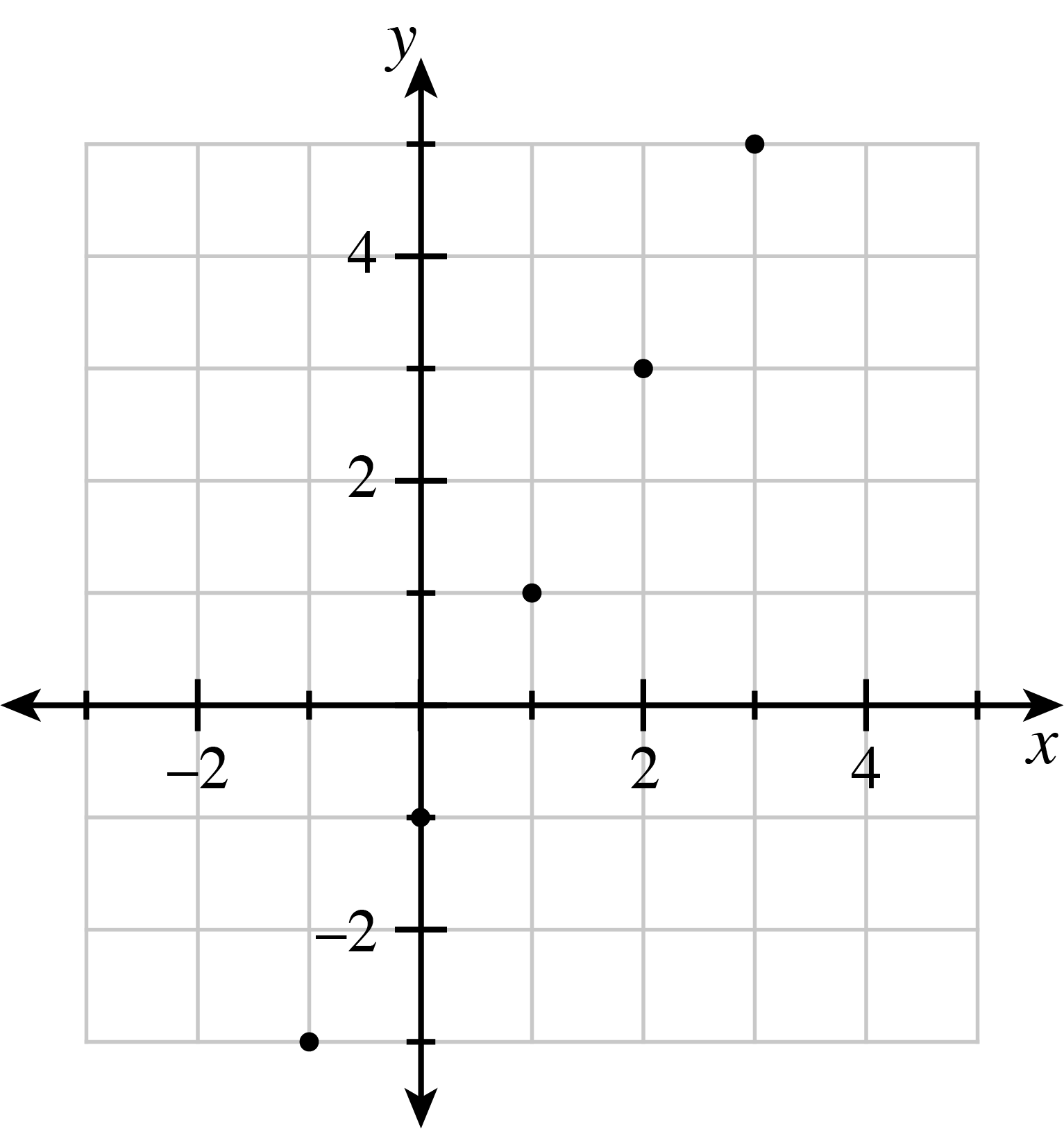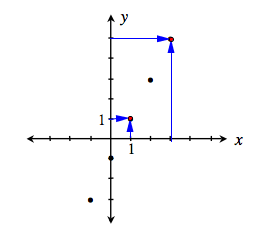### Home > CC3 > Chapter 3 > Lesson 3.2.2 > Problem3-85

3-85.Examine the graph at right.

1. Use the graph to complete the table:IN (x) OUT (y) $\color{blue}{1}$ $\color{blue}{3}$ $\color{blue}{1}$ $\color{blue}{5}$
2. Use the graph to find the rule:
$y=$ ___________

The graph is a line, so use what you know about rules in tables.